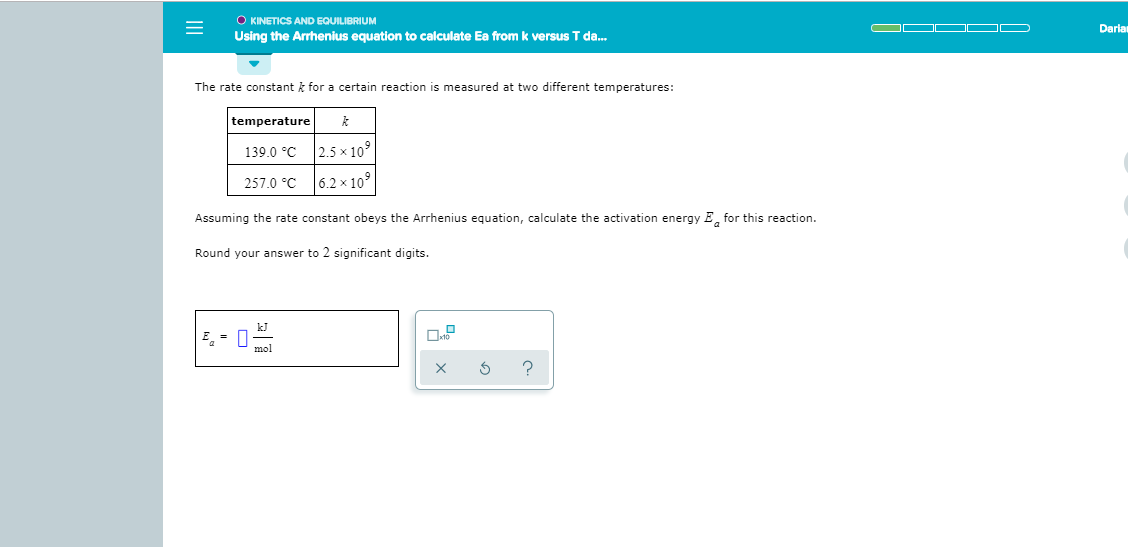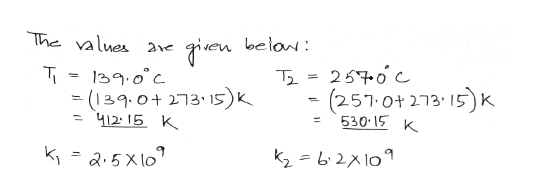O KINETICS AND EQUILIBRIUMOO DODariaUsing the Arrhenius equation to calculate Ea from k versus T d...The rate constant k for a certain reaction is measured at two different temperatures:temperature2.5x 109139.0 C6.2 x 109257.0 CAssuming the rate constant obeys the Arrhenius equation, calculate the activation energy E for this reactionRound your answer to 2 significant digits.kJmolX

Questionhelp_outlineImage TranscriptioncloseO KINETICS AND EQUILIBRIUM OO D O Daria Using the Arrhenius equation to calculate Ea from k versus T d... The rate constant k for a certain reaction is measured at two different temperatures: temperature 2.5x 109 139.0 C 6.2 x 109 257.0 C Assuming the rate constant obeys the Arrhenius equation, calculate the activation energy E for this reaction Round your answer to 2 significant digits. kJ mol X fullscreen
Step 1help_outlineImage TranscriptioncloseThe values en belan: 250 c 139.0c = (139.0+ 273 5) 41215 K (257.0t 273 15K 530 15 K K2= b210 fullscreen

Want to see the full answer?

See Solution

Want to see this answer and more?

Our solutions are written by experts, many with advanced degrees, and available 24/7

See Solution
Tagged in

Chemistry